• 累计撰写 145 篇文章
• 累计创建 86 个标签
• 累计收到 25 条评论

### 目 录CONTENT# Pony有个性的ORM框架！2022-06-18 / 0 评论 / 3 点赞 / 505 阅读 / 4,646 字 / 正在检测是否收录...

## 2、快速开始

### 2.1、安装Pony

pip install pony


### 2.2、链接数据库

from pony.orm import *

db = Database()
db.bind('sqlite','mydb.db',create_db=True)


### 2.3、创建模型类（表）

from pony.orm import *

db = Database()
db.bind('sqlite','mydb.db',create_db=True)

# 定义一个表：person
class Person(db.Entity):
_table_ = 'person' # 表名
# 当我们不定义主键ID的时候，pony会和大多数orm框架一样自动帮我们补全
# id = PrimaryKey(int, auto = True)
name = Required(str, 30, default = "未知")
age = Optional(int)
car = Set('Car') # 以集合的形式关联Car表，即一个人多个车

class Car(db.Entity):
_table_ = 'car'
name = Required(str)
typa = Required(str)
owner = Required(Person) # 关联Person表，即一个车的主人只有一个

if __name__ == '__main__':
# 1.查看表结构
show(Car)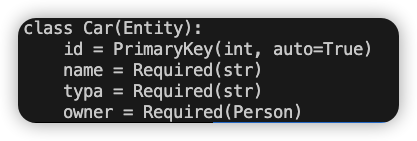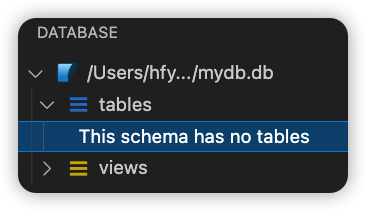### 2.4、将表映射到数据库

if __name__ == '__main__':
# 1.查看表结构
# show(Car)

# 2.将模型类映射到数据库，以表的形式展现
db.generate_mapping(create_tables=True) # 如果表存在则对表结构进行更新，如果不存在则创建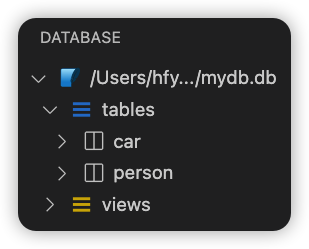### 2.5、CRUD之C

...
@db_session
def create_person(name,age):
perObj = Person(name=name,age=age)
...

if __name__ == '__main__':

# 3.CRUD之C
db.generate_mapping(create_tables=True)
create_person('phyger',18)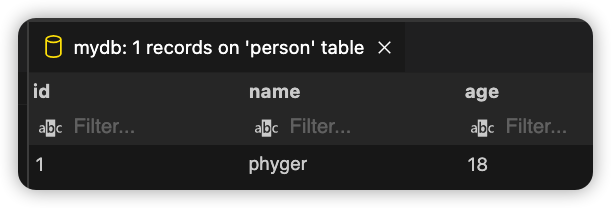### 2.6、CRUD之R

...
@db_session
def get_person(name):
persObj = Person.select(name=name)
print(persObj.first().age)
...
if __name__ == '__main__':

# 4.CRUD之R
db.generate_mapping(create_tables=True)
get_person('phyger')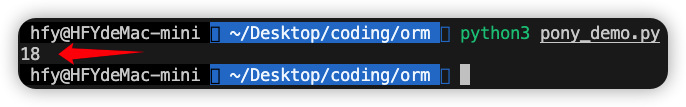### 2.7、CRUD之U

...
@db_session
def put_person(name,new_name=None,new_age=None):
persObj = Person.select(name=name)
if new_name:
persObj.first().name=new_name
if new_age:
persObj.first().age=new_age
print('更新成功！')
...
if __name__ == '__main__':

# 5.CRUD之U
db.generate_mapping(create_tables=True)
get_person('phyger')
put_person('phyger',new_name='new_phyger')
get_person('phyger')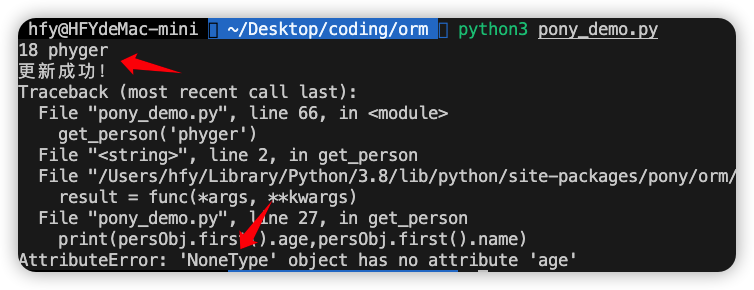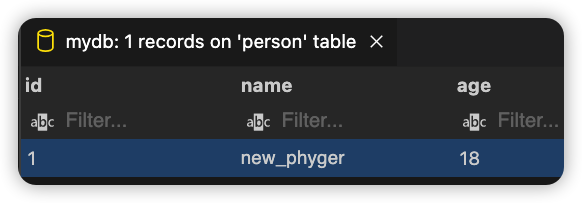### 2.8、CRUD之D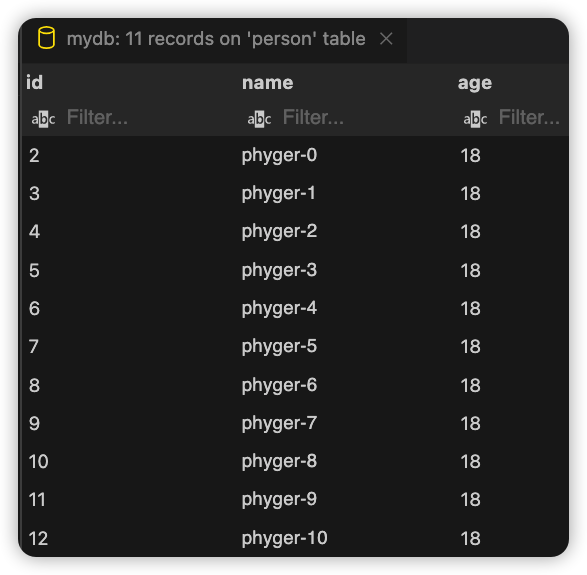...
@db_session
def del_person(name):
delete(p for p in Person if p.name==name) # 根据名称进行删除
...
if __name__ == '__main__':

# 6.CRUD之D
db.generate_mapping(create_tables=True)
del_person('phyger-8')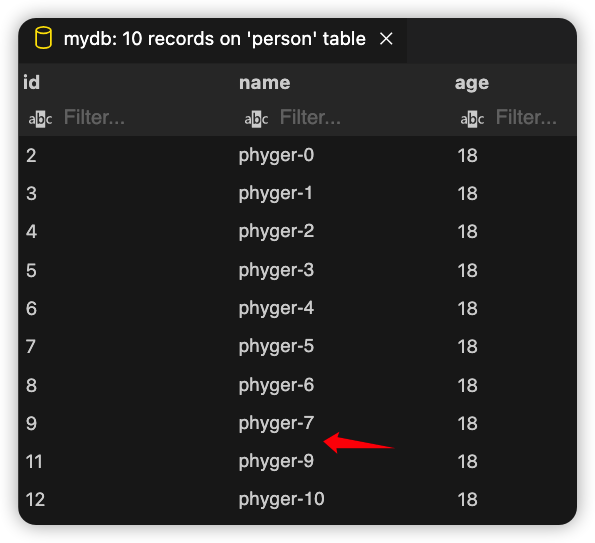## 3、进阶

### 3.1、完善查询

@db_session
def get_person(name=None,id=None,age=None):
if name:
for p in Person.select(name=name):
print(p.id,p.name)
if id:
for p in Person.select(id=id):
print(p.id,p.name)
if age:
for p in Person.select(age=age):
print(p.id,p.name)


### 3.2、批量删除

@db_session
def del_person(name=None,idd=None,age=None):
if name:
delete(p for p in Person if p.name==name)
if idd:
delete(p for p in Person if p.id==idd)
if age:
delete(p for p in Person if p.age==age)
if __name__ == '__main__':

# 批量删除
db.generate_mapping(create_tables=True)
del_person(age=18)


### 3.4、分页

@db_session
def person_page(num,size):
PObj=Person.select()
for p in PObj.page(num,size):
print(p.name)
if __name__ == '__main__':

# 7.分页
db.generate_mapping(create_tables=True)
person_page(1,5)  # 第一页，单页大小5   0到4
person_page(2,3)  # 第二页，单页大小3   3,4,5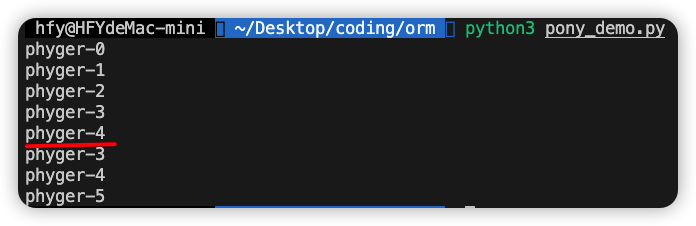### 3.5、表关联

@db_session
def create_car(name, typa, owner=None):
if owner:
carObj = Car(name=name, typa=typa, owner=owner)
else:
carObj = Car(name=name, typa=typa)

if __name__ == '__main__':
# 8、创建Car-1
db.generate_mapping(create_tables=True)
create_car(name='byd',typa='宋Pro-Dmi')


if __name__ == '__main__':

# 8、创建Car-2
db.generate_mapping(create_tables=True)
pp = get_person(id=14)
print(pp)
create_car(name='吉利',typa='星越L',owner=pp.id)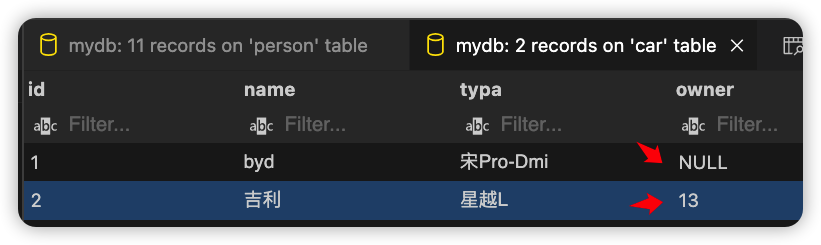@db_session
def get_car(name=None, id=None, owner=None):
if name:
for c in Car.select(name=name):
print(c.id, c.name)
if id:
for c in Car.select(id=id):
print(c.id, c.name)
if owner:
for c in Car.select(owner=owner):
print(c.id, c.name)

if __name__ == '__main__':

# 9、表关联的查询
db.generate_mapping(create_tables=True)
ps = get_person(name='phyger-0')
get_car(owner=ps.id)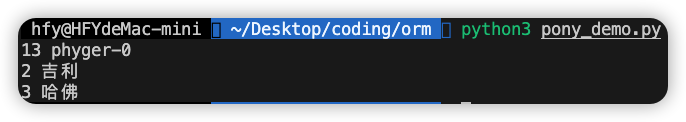Car数据表内容如下：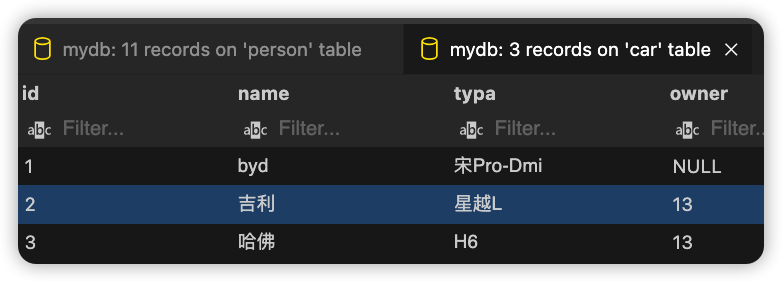## 4、最后

3
1.2.• 3
1.2.